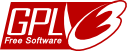As a new sequel to the Excel random password generator and the Random password generator, I’ve made a PHP random password generator service…

You can call it this way (if you hover the parameter values, you’ll get an explanation):

`http://www.neoegm.com/services/random_password.php?length=8&upper=1&lower=1&numbers=1`

So, for example, to generate a 10 characters numeric password you could access:

`http://www.neoegm.com/services/random_password.php?length=10&upper=0&lower=0`

That’s all, now the code for the ones who want to use it on their own sites (read below for license)…The code is licensed under the GNU GPL v3

Anyway, here is the code so you can watch it without having to download the PHP file:

```function make_seed()	//Function make_seed from http://www.php.net/manual/en/function.srand.php
{
list(\$usec, \$sec) = explode(' ', microtime());
return (float) \$sec + ((float) \$usec * 100000);
}

function RandomPassword(\$length, \$upper = true, \$numbers = true, \$lower = true)
{
if (!\$upper && !\$lower && !\$numbers)
return "";

\$ret = "";

\$chars = 26 * 2 + 10;	//26 (a-z) + 26 (A-Z) + 10 (0-9)
//a-z = 97-122
//A-Z = 65-90
//0-9 = 48-57

srand(make_seed());		//Seed with microseconds
//if you don't need this, you can just use srand(time());

for (\$i = 1; \$i <= \$length; \$i++)
{
\$repeat = false;

\$num = floor(rand(0, \$chars-1));

if (\$num < 26)
if (\$lower)
\$ret .= chr(\$num + 97);
else
\$repeat = true;
else if (\$num < 52)
if (\$upper)
\$ret .= chr(\$num - 26 + 65);
else
\$repeat = true;
else if (\$num < 62)
if (\$numbers)
\$ret .= chr(\$num - 52 + 48);
else
\$repeat = true;

if (\$repeat)
\$i--;
}

return \$ret;
}
```

And this is the code needed to make it a web service:

```\$length = isset(\$_REQUEST['length'])?\$_REQUEST['length']:8;
\$upper = isset(\$_REQUEST['upper'])?\$_REQUEST['upper']:true;
\$numbers = isset(\$_REQUEST['numbers'])?\$_REQUEST['numbers']:true;
\$lower = isset(\$_REQUEST['lower'])?\$_REQUEST['lower']:true;

if (\$length < 1 || \$length > 128)
\$length = 8;

if (!(\$upper == false || \$upper == 0))
\$upper = true;

if (!(\$numbers == false || \$numbers == 0))
\$numbers = true;

if (!(\$lower == false || \$lower == 0))
\$lower = true;

```

### Support appreciated!

All the content offered in this website is, except noted otherwise, of free nature. This means you can share it wherever you want if you do it freely and stating its source.

If it was useful for you and you’d like to contribute, you can make a donation or, at least, visit one of our advertisers of your choice; they are all around the site.

#### 2 Responses to “PHP random password generator”

•Eremeeff

Hi,
Thanks for article. Everytime like to read you.
Thanks

•NeoEGM

Thanks to you Eremeeff!